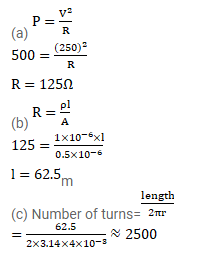# A heater-coil is to be constructed with a nichrome wire

Question:

A heater-coil is to be constructed with a nichrome wire $\left(\rho=1.0 \times 10^{-6} \Omega-\mathrm{m}\right)$ which can operate at $500 \mathrm{~W}$ when connected to a $250 \mathrm{~V}$ supply. (a) What would be the resistance of the coil? (b) If the cross-sectional area of the wire is $0.5 \mathrm{~mm}{ }^{2}$, what length of the wire will be needed? (c) If the radius of each turn is $4.0 \mathrm{~mm}$, how many turns will be there in the coil?

Solution: